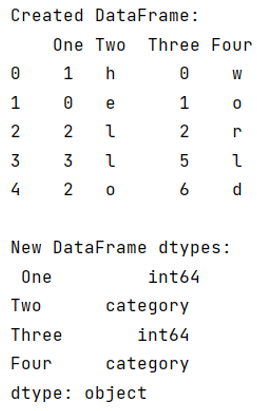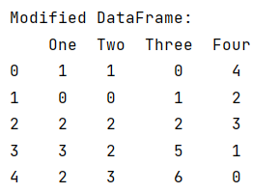# Convert categorical data in pandas dataframe

Given a Pandas DataFrame, we have to convert categorical data in it. By Pranit Sharma Last updated : September 23, 2023

Pandas is a special tool that allows us to perform complex manipulations of data effectively and efficiently. Inside pandas, we mainly deal with a dataset in the form of DataFrame. DataFrames are 2-dimensional data structures in pandas. DataFrames consist of rows, columns, and the data. The Data inside the DataFrame can be of any type.

## Problem statement

Given a Pandas DataFrame, we have to convert categorical data in it.

## Converting categorical data in pandas dataframe

Categorical data is a type of data that has some certain category or characteristic, the value of categorical data is not a single value, rather it consists of classified values, for example, an email can be considered as spam or not spam, if we consider 1 as spam and 0 as not spam, we have a classified data in the form of 0 or 1, this is called categorical data. We will pass a string called 'category' inside the astype() method to first make the data categorical.

Note

To work with pandas, we need to import pandas package first, below is the syntax:

```import pandas as pd
```

Let us understand with the help of an example,

## Python program to convert categorical data in pandas dataframe

```# Importing pandas package
import pandas as pd

# Creating a dictionary
d = {
'One':[1,0,2,3,2],
'Two':list('hello'),
'Three':[0,1,2,5,6],
'Four':list('world')
}

# Creating dataframe
df = pd.DataFrame(d)

# Display DataFrame
print("Created DataFrame:\n",df,"\n")

# Changing dtypes of column Two and Four
df['Two'] = df['Two'].astype('category')
df['Four'] = df['Four'].astype('category')

# Display dtypes of df
print("New DataFrame dtypes:\n",df.dtypes,"\n")
```

### Output

The output of the above program is:## Select all those columns whose data type is categorical and then use cat.codes() method

```# Changing dtypes of column Two and Four
df['Two'] = df['Two'].astype('category')
df['Four'] = df['Four'].astype('category')

# Display dtypes of df
print("New DataFrame dtypes:\n",df.dtypes,"\n")

# Selecting columns having dtpe category
category = df.select_dtypes(['category']).columns

# Converting category data into df
df[category] = df[category].apply(lambda x: x.cat.codes)

# Display modified DataFrame
print("Modified DataFrame:\n",df)
```

### Output

The output of the above program is: